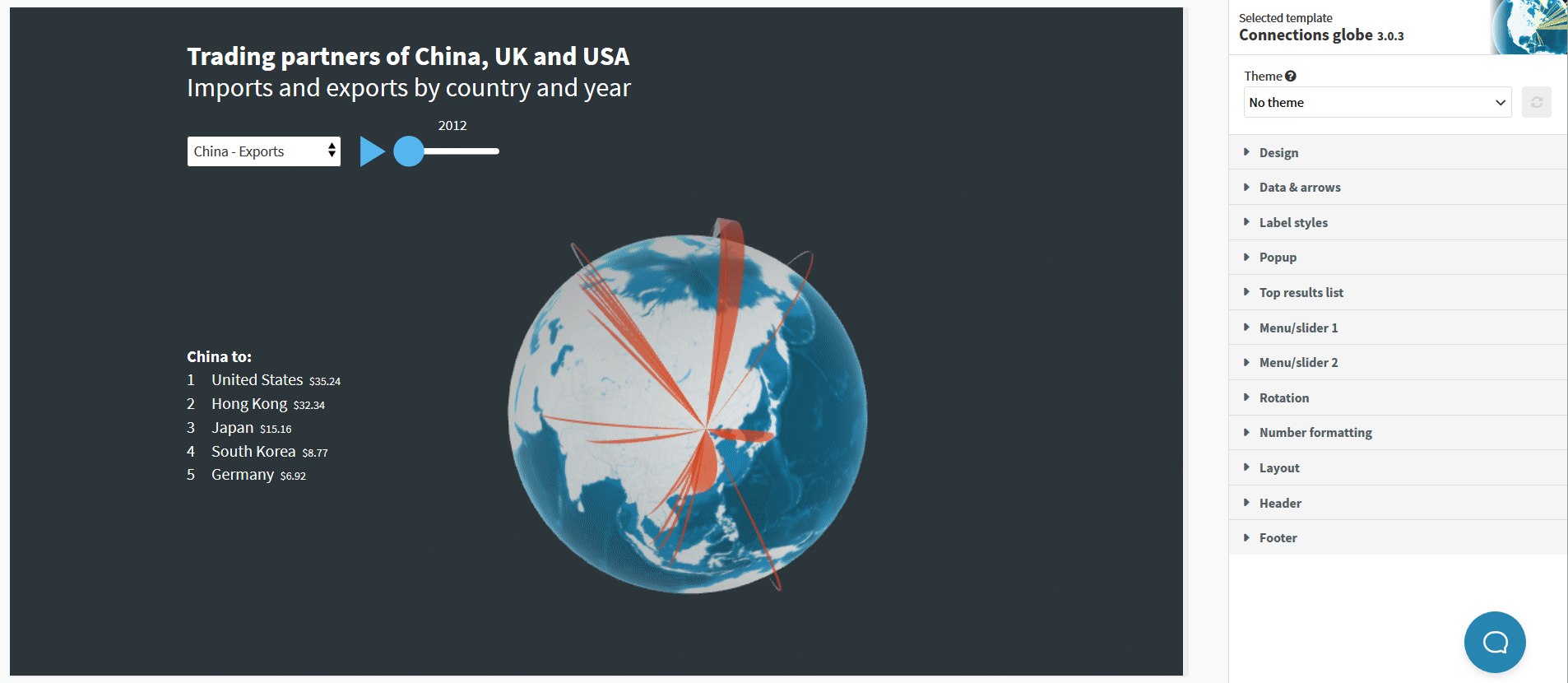# Sum, maximum, mean or median? How to handle values

In your datasheet there may be only one value for rows with the same origin and destination. However, this is often not the case. What if you need to only show the highest of a range of values, or calculate their sum, mean or median?

1
Head over to Preview and then to the Data and Arrows options.
2
From the dropdown menu, you are able to choose between summing the values, pick out the maximum or the minimum, calculate the mean or the median, or alternatively count them.TIP:: The mean is the sum of the numbers in the set divided by their amount.

The  median is the middle point of a value set, in which half the values are above the median number and half are below. If your value set features an even number of numbers you are able to calculate the median by the two middle numbers and dividing by 2.